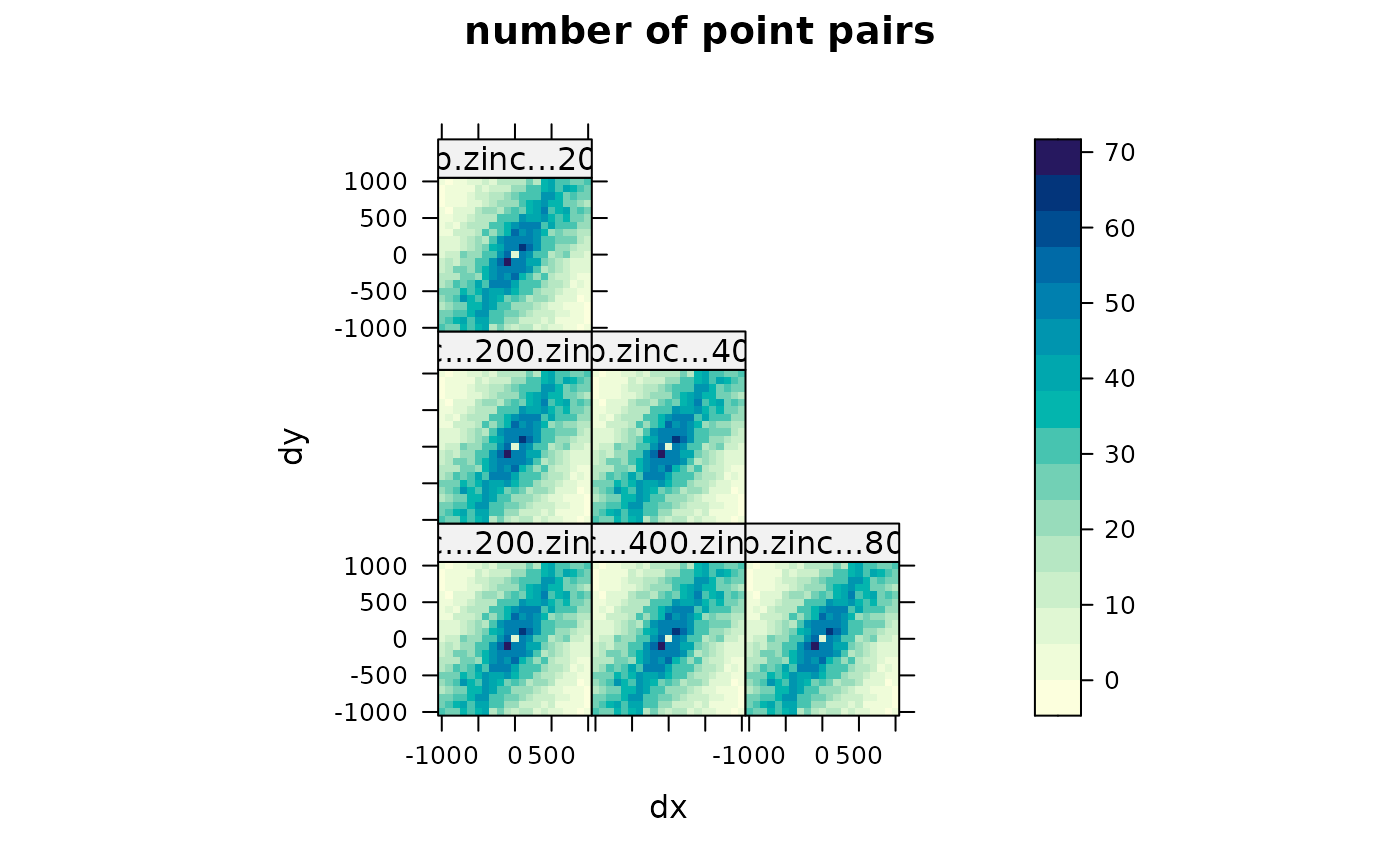Creates a variogram plot

# S3 method for gstatVariogram
plot(x, model = NULL, ylim, xlim, xlab = "distance",
ylab = attr(x, "what"), panel = vgm.panel.xyplot, multipanel = TRUE,
plot.numbers = FALSE, scales, ids = x\$id, group.id = TRUE, skip,
layout, ...)
# S3 method for variogramMap
plot(x, np = FALSE, skip, threshold, ...)
# S3 method for StVariogram
plot(x, model = NULL, ..., col = bpy.colors(), xlab, ylab,
map = TRUE, convertMonths = FALSE, as.table = TRUE, wireframe = FALSE,
diff = FALSE, all = FALSE)

## Arguments

x object obtained from the method variogram, possibly containing directional or cross variograms, space-time variograms and variogram model information in case of a single variogram: a variogram model, as obtained from vgm or fit.variogram, to be drawn as a line in the variogram plot; in case of a set of variograms and cross variograms: a list with variogram models; in the spatio-temporal case, a single or a list of spatio-temporal models that will be plotted next to each other for visual comparison. numeric; vector of length 2, limits of the y-axis numeric; vector of length 2, limits of the x-axis character; x-axis label character; y-axis label panel function logical; if TRUE, directional variograms are plotted in different panels, if FALSE, directional variograms are plotted in the same graph, using color, colored lines and symbols to distinguish them logical or numeric; if TRUE, plot number of point pairs next to each plotted semivariance symbol, if FALSE these are omitted. If numeric, TRUE is assumed and the value is passed as the relative distance to be used between symbols and numeric text values (default 0.03). optional argument that will be passed to xyplot in case of the plotting of variograms and cross variograms; use the value list(relation = "same") if y-axes need to share scales ids of the data variables and variable pairs logical; control for directional multivariate variograms: if TRUE, panels divide direction and colors indicate variables (ids), if FALSE panels divide variables/variable pairs and colors indicate direction logical; can be used to arrange panels, see xyplot integer vector; can be used to set panel layout: c(ncol,nrow) logical (only for plotting variogram maps); if TRUE, plot number of point pairs, if FALSE plot semivariances semivariogram map values based on fewer point pairs than threshold will not be plotted any arguments that will be passed to the panel plotting functions (such as auto.key in examples below) colors to use logical; if TRUE, plot space-time variogram map logical; if TRUE, yearmon time lags will be unit converted and plotted as (integer) months, and no longer match the numeric representation of yearmon, which has years as unit controls the plotting order for multiple panels, see xyplot for details. logical; if TRUE, produce a wireframe plot logical; if TRUE, plot difference between model and sample variogram; ignores all. logical; if TRUE, plot sample and model variogram(s) in single wireframes.

## Value

returns (or plots) the variogram plot

## Details

Please note that in the spatio-temporal case the levelplot and wireframe plots use the spatial distances averaged for each time lag avgDist. For strongly varying spatial locations over time, please check the distance columns dist and avgDist of the spatio-temporal sample variogram. The lattice::cloud function is one option to plot irregular 3D data.

http://www.gstat.org

Edzer Pebesma

## Note

currently, plotting models and/or point pair numbers is not supported when a variogram is both directional and multivariable; also, three-dimensional directional variograms will probably not be displayed correctly.

## Examples

library(sp)
data(meuse)
coordinates(meuse) = ~x+y
vgm1 <- variogram(log(zinc)~1, meuse)
plot(vgm1)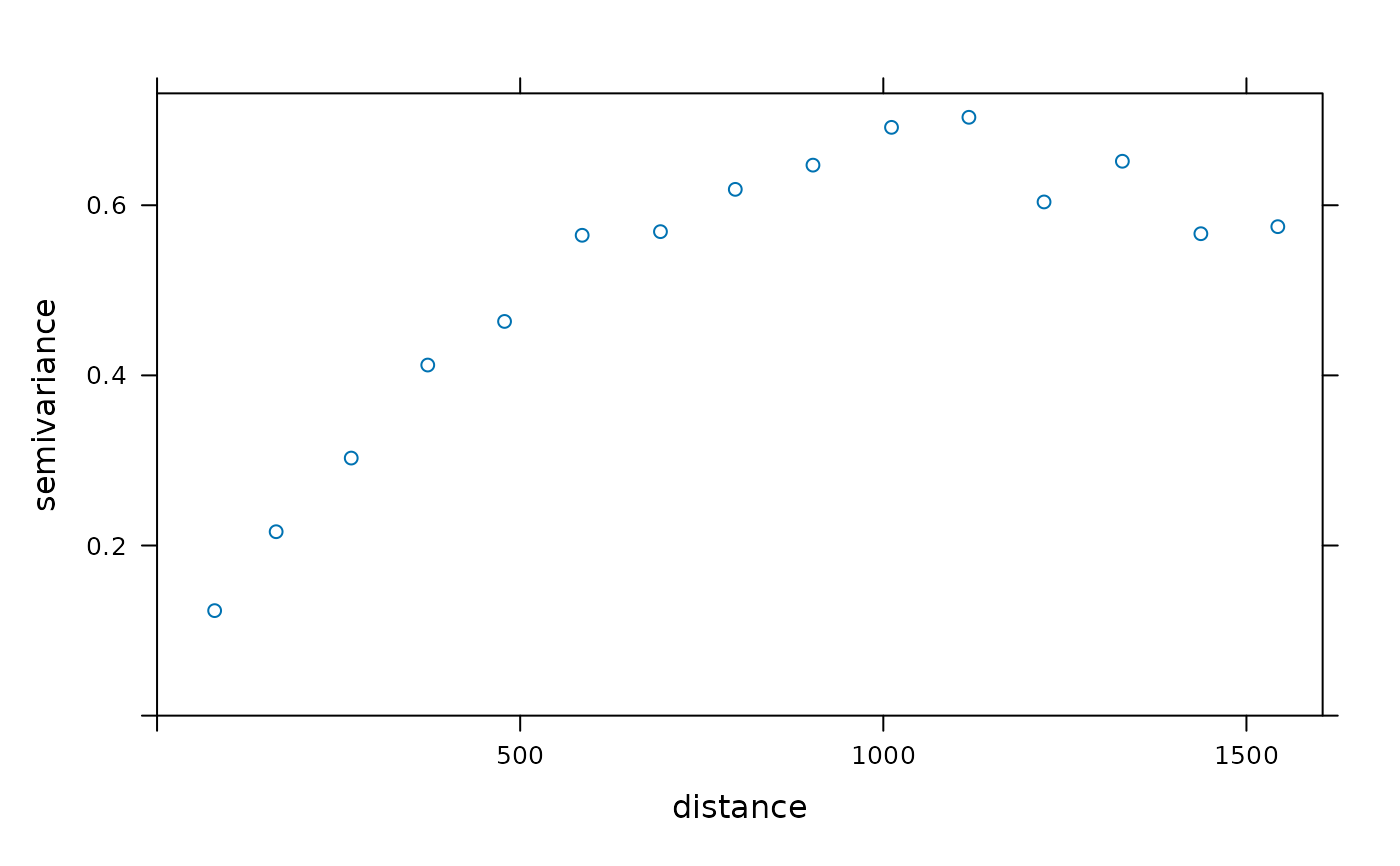model.1 <- fit.variogram(vgm1,vgm(1,"Sph",300,1))
plot(vgm1, model=model.1)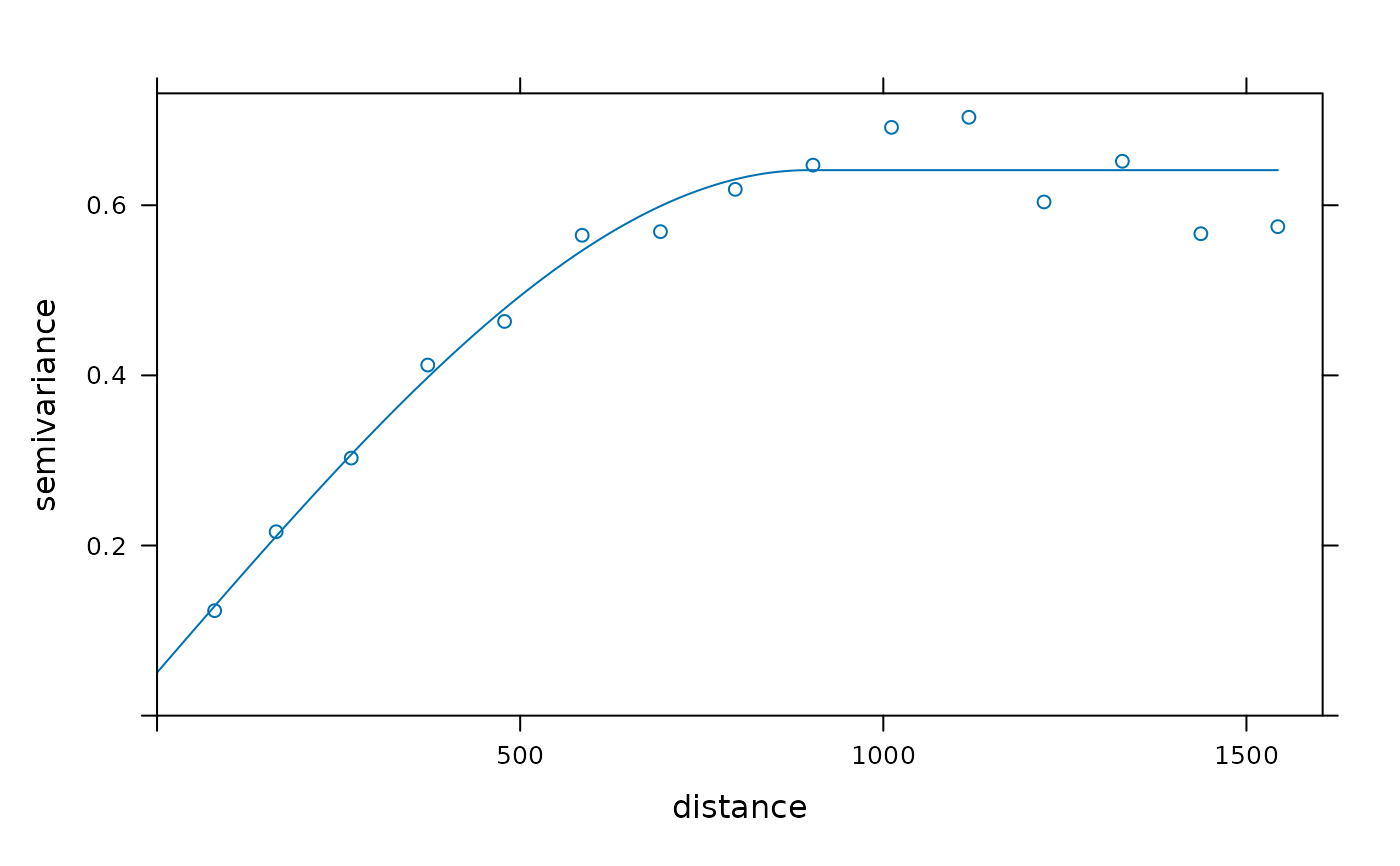plot(vgm1, plot.numbers = TRUE, pch = "+")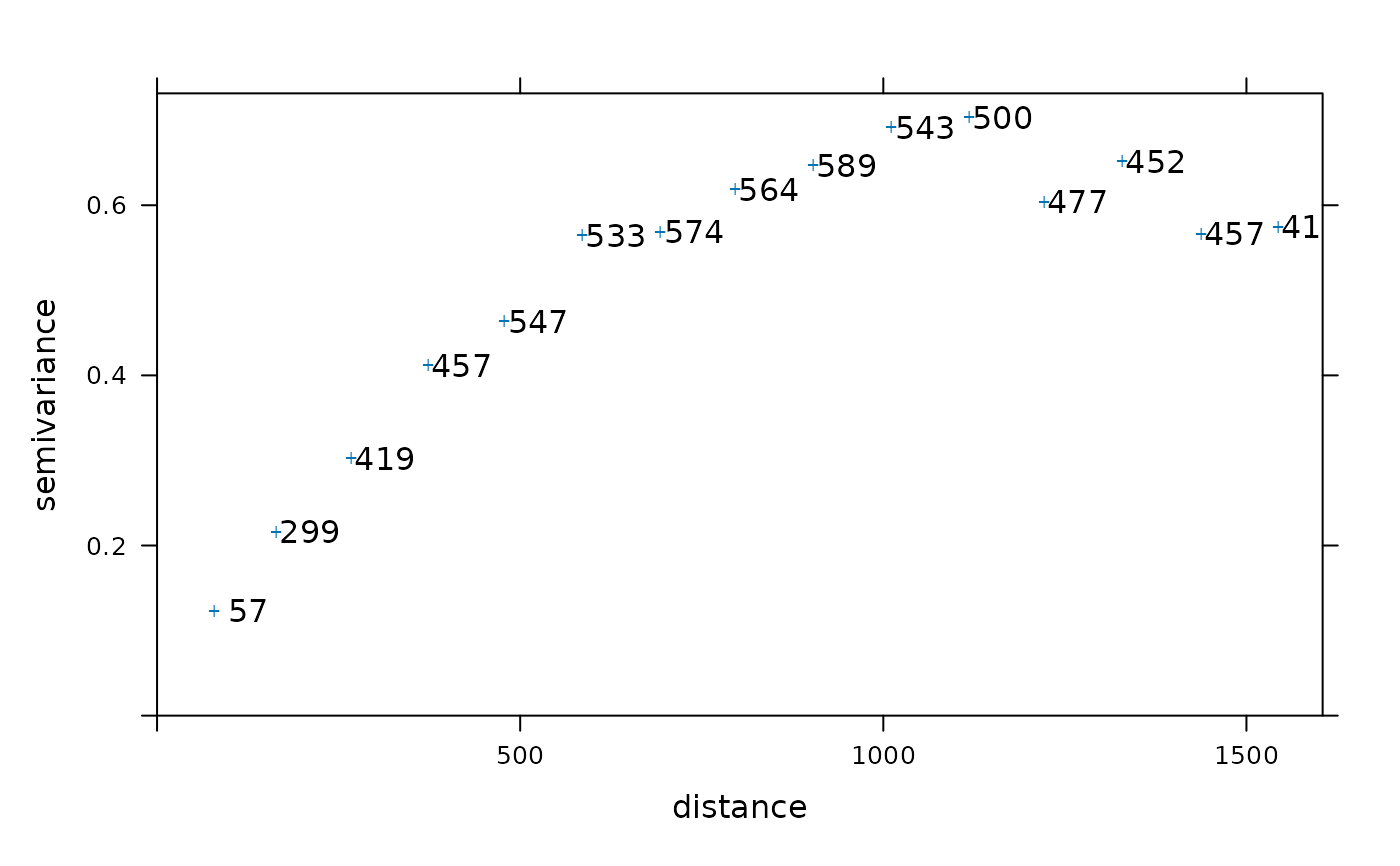vgm2 <- variogram(log(zinc)~1, meuse, alpha=c(0,45,90,135))
plot(vgm2)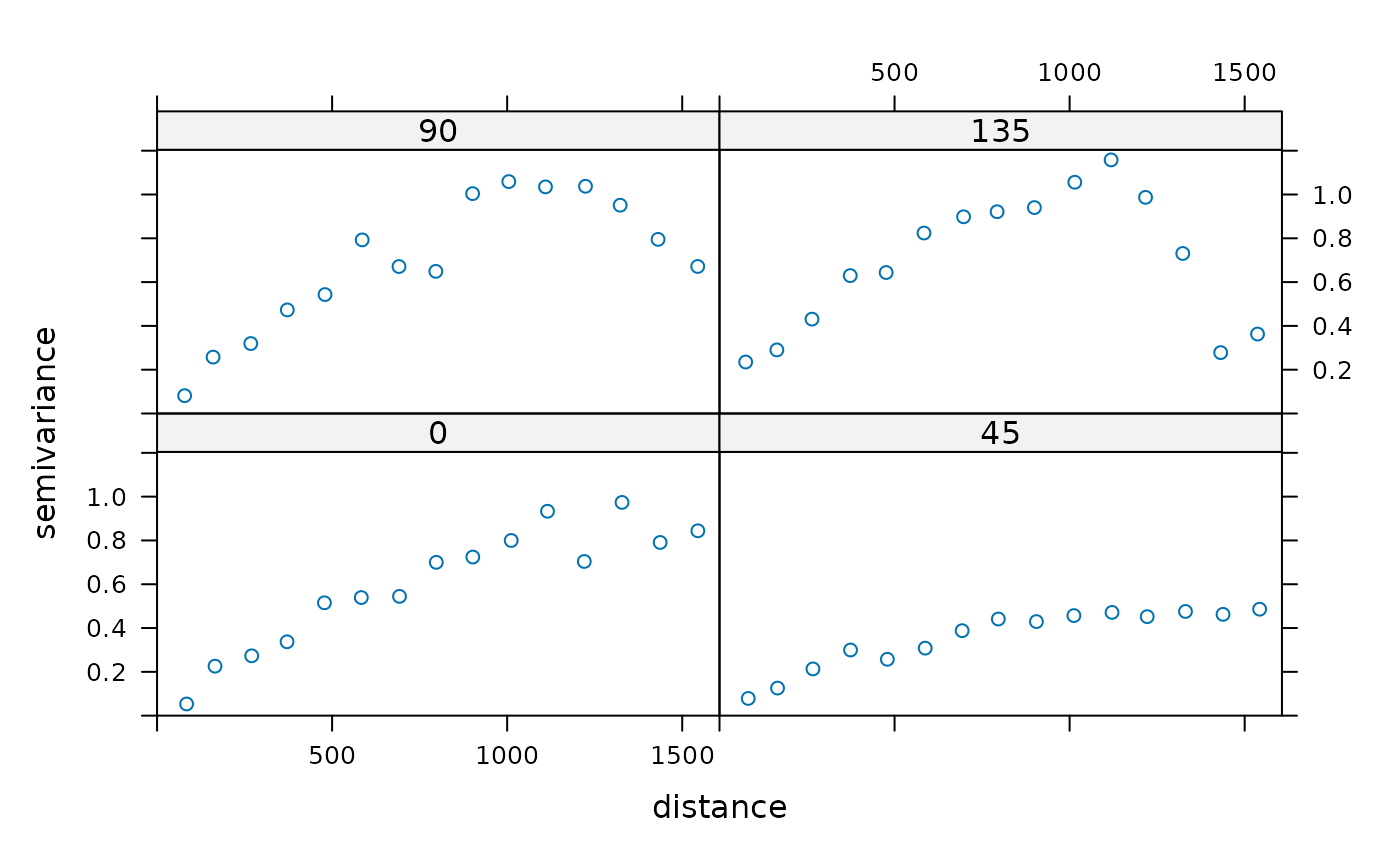# the following demonstrates plotting of directional models:
model.2 <- vgm(.59,"Sph",926,.06,anis=c(0,0.3))
plot(vgm2, model=model.2)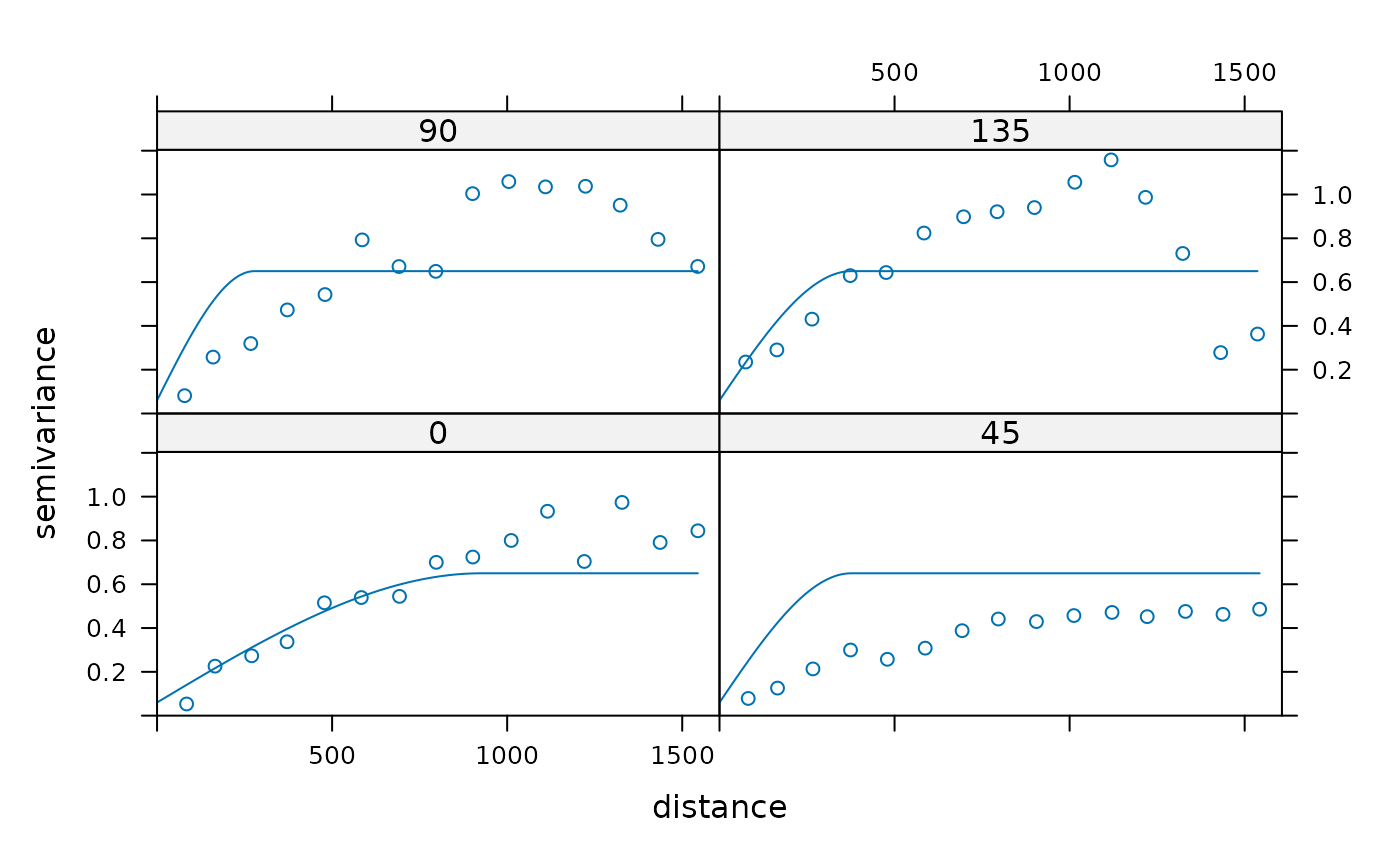g = gstat(NULL, "zinc < 200", I(zinc<200)~1, meuse)
g = gstat(g, "zinc < 400", I(zinc<400)~1, meuse)
g = gstat(g, "zinc < 800", I(zinc<800)~1, meuse)
# calculate multivariable, directional variogram:
v = variogram(g, alpha=c(0,45,90,135))
plot(v, group.id = FALSE, auto.key = TRUE) # id and id pairs panels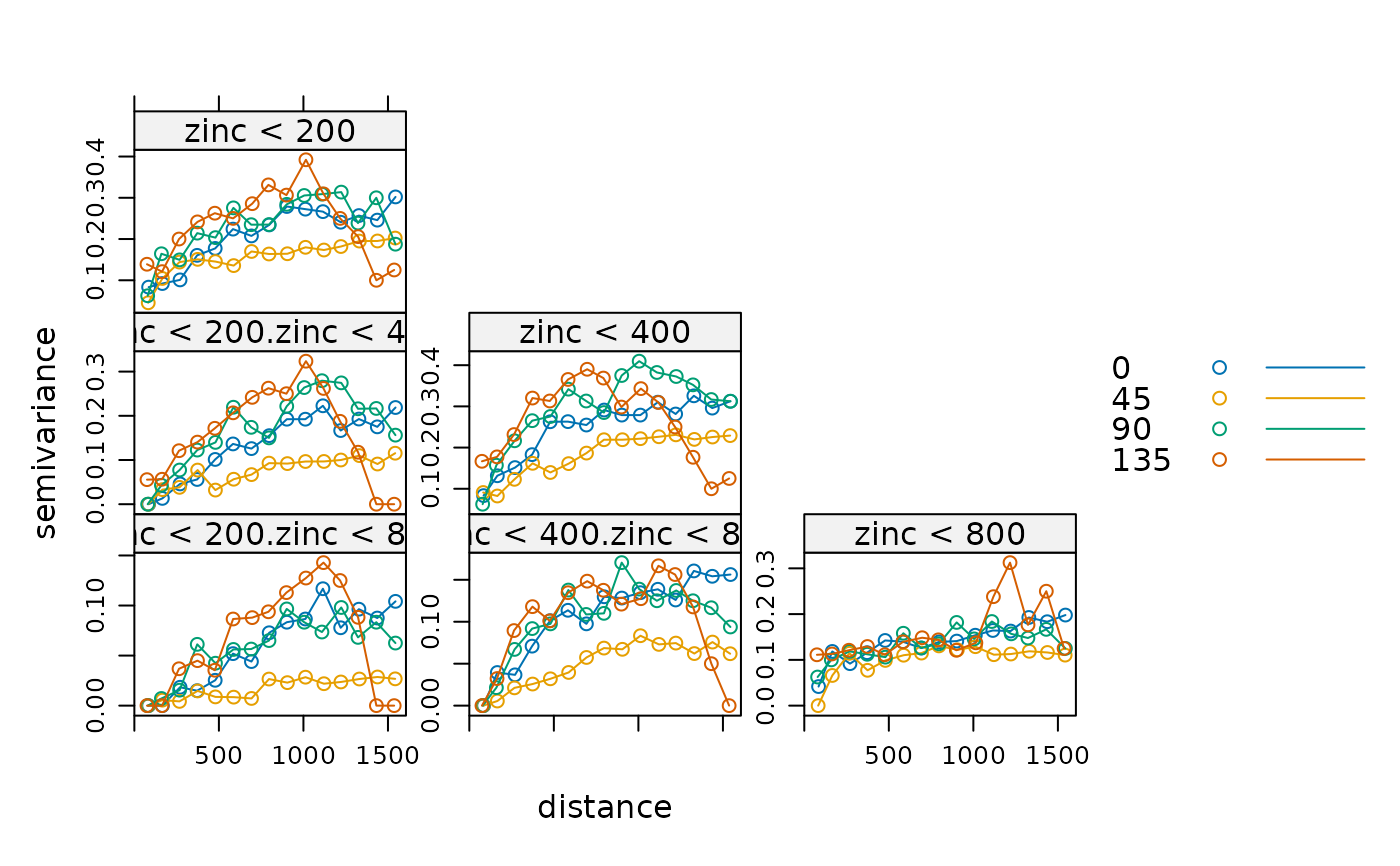plot(v, group.id = TRUE, auto.key = TRUE)  # direction panels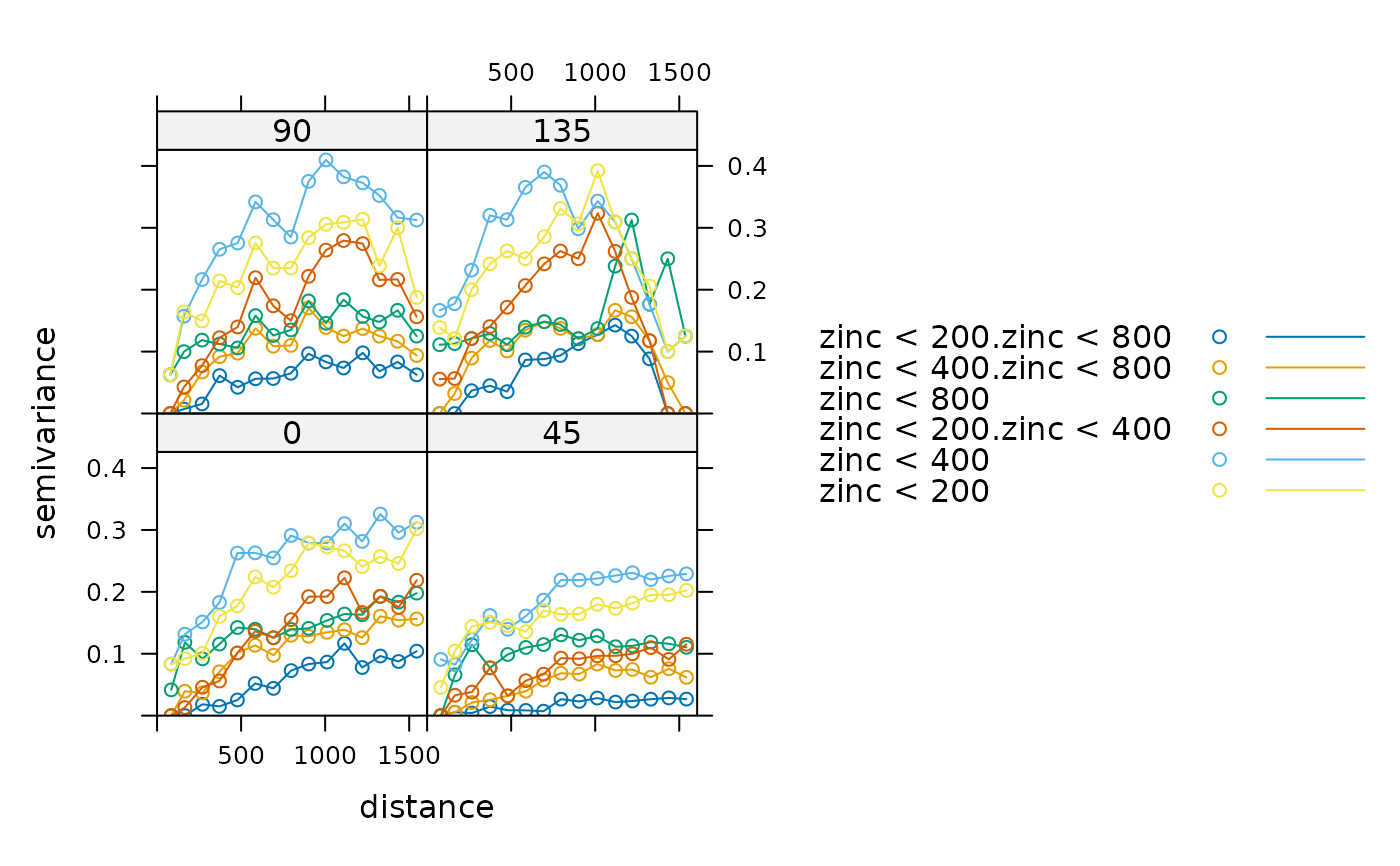# variogram maps:
plot(variogram(g, cutoff=1000, width=100, map=TRUE),
main = "(cross) semivariance maps")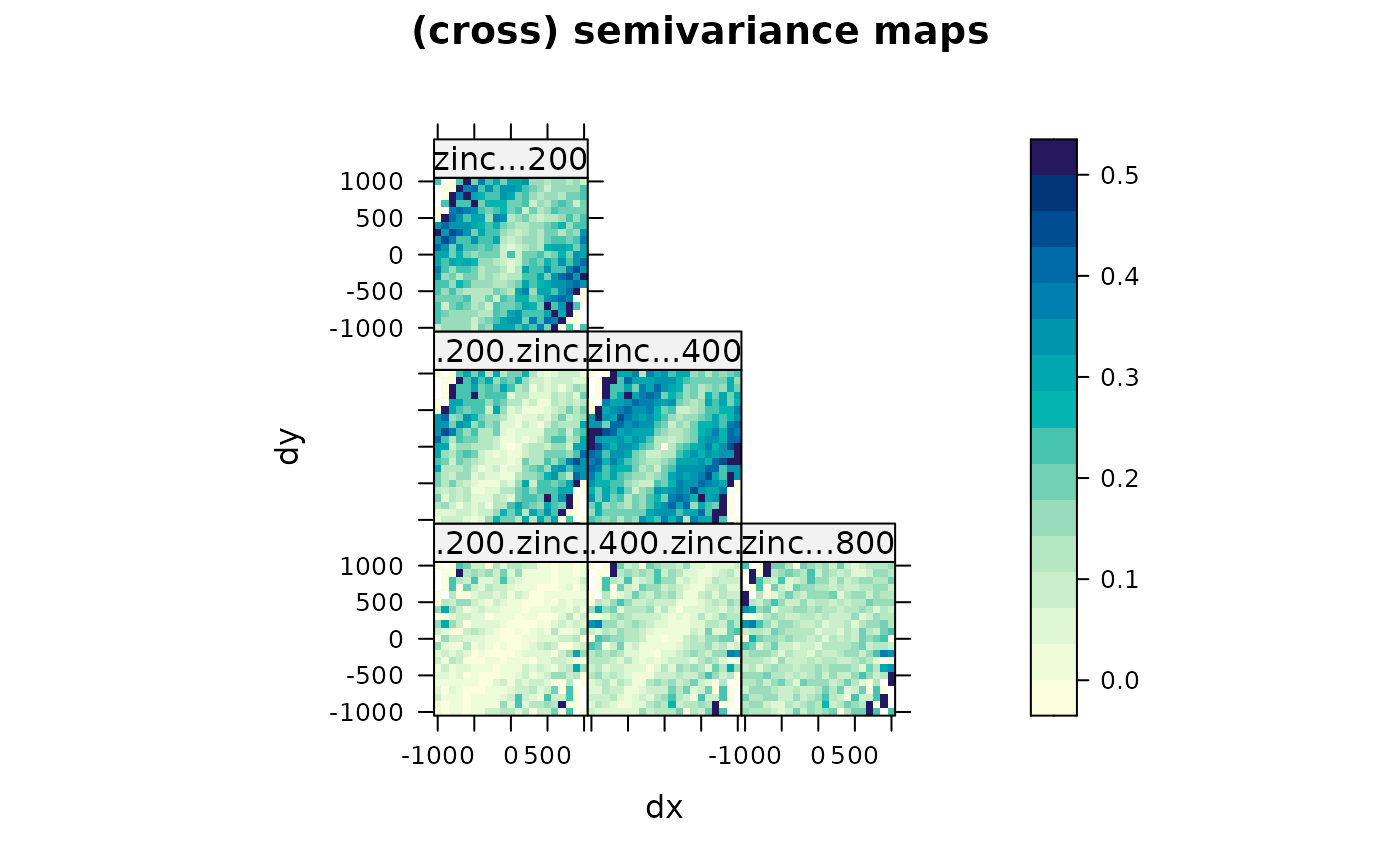plot(variogram(g, cutoff=1000, width=100, map=TRUE), np=TRUE,
main = "number of point pairs")